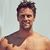# Can You Score 15/15 In This Impossible Math Quiz?

## This impossible math quiz has been driving the internet crazy.Created by Michael Rogers
On Aug 17, 2021

We gave this math quiz to a group of 120 college students and no one got a perfect score. Can you?

1 / 13

What is the square root of 4,096?

2 / 13

4 + (8 – 4) 4 = ?

3 / 13

When you add the square root of 100 to 100 squared, what do you get?

4 / 13

3 − 3 + 3 × 3 = ?

5 / 13

25 x 25 = ?

6 / 13

Pick the dimensions of a square whose perimeter and area are EQUAL in value?

7 / 13

6 ÷ 2 (2 + 1) = ?

8 / 13

1 + 1 ÷ 1 + 1 x 1 = ?

9 / 13

Which is divisible by 7 without a remainder?

10 / 13

4 + 2 (8 – 6) = ?

11 / 13

Convert five percent to decimals

12 / 13

If X is bigger than Y but smaller than Z, then...

13 / 13

9 ÷ 3 + 4 (11 − 5) = ?

13
Questions left

### How much did you like this quiz?

Calculating results